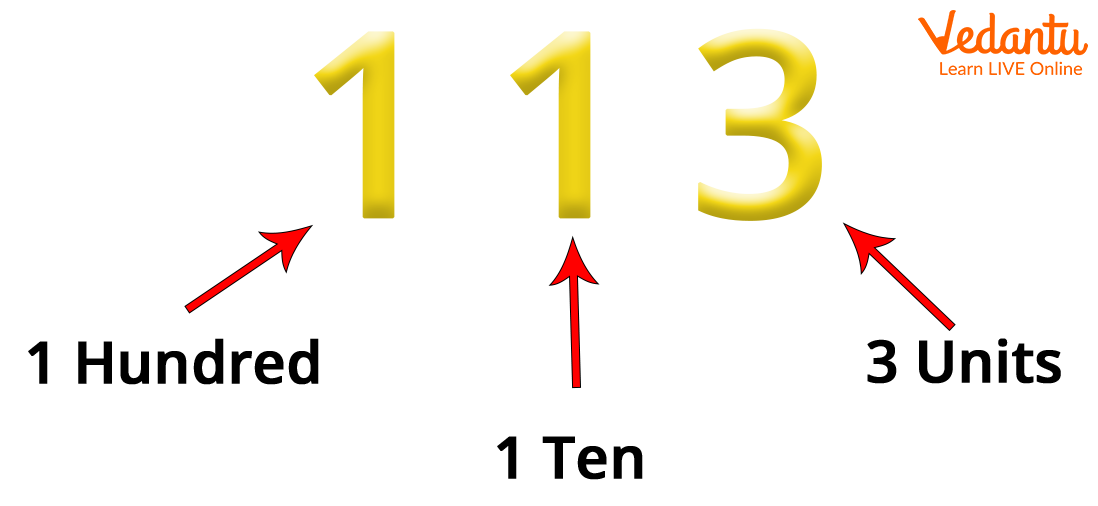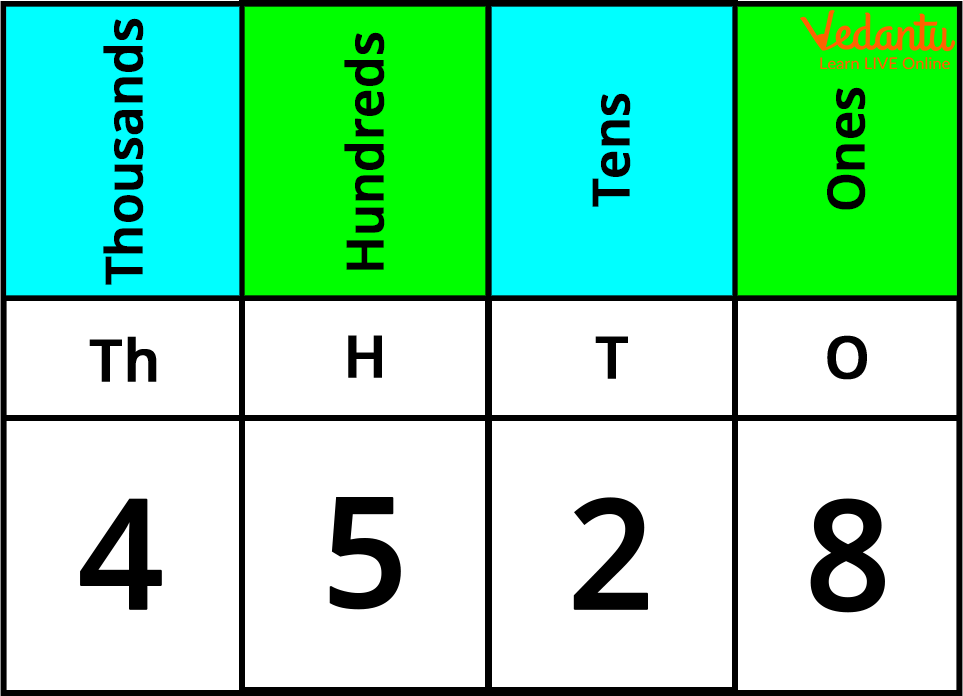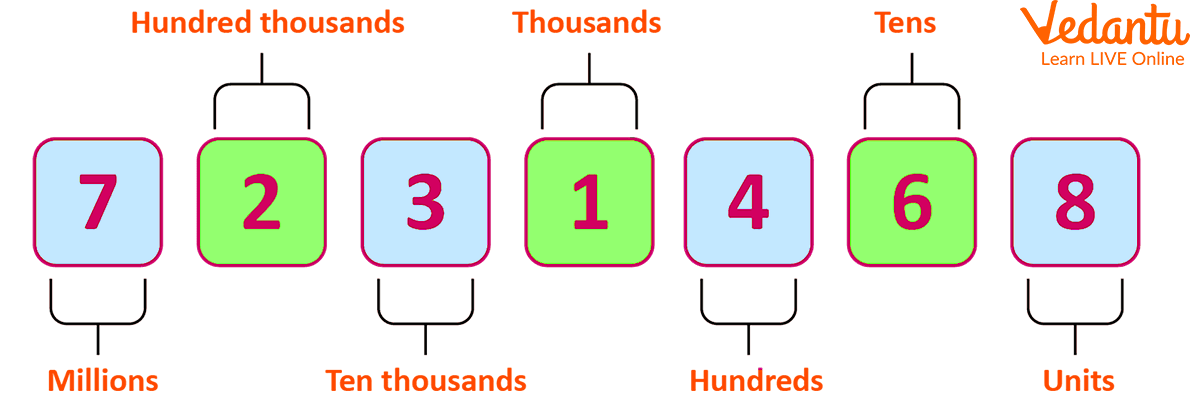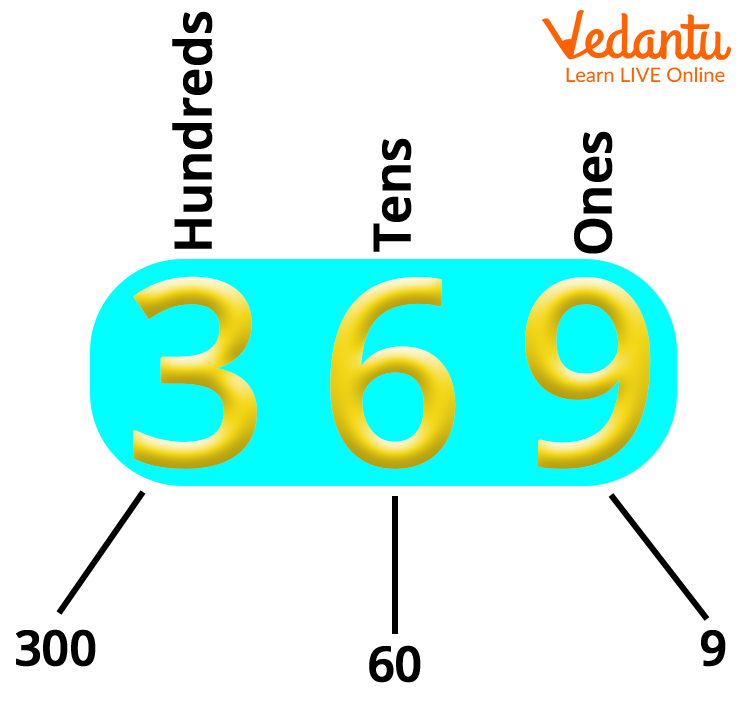Courses
Courses for Kids
Free study material
Free LIVE classes
More

# The Concept of Place Value in Year 3## An Introduction to Place Value

In Year 3 Maths, kids generally learn to use the place value to identify the place value of digits, they are also taught to use the place value chart. Teaching your kids about the use and concept of place value can help them develop a better understanding of Maths concepts. Understanding place value can be easier when explained with various pictorial representations. Here, we have tried explaining the meaning of the place value, along with some tips that can help teachers and parents to help kids understand the concept.

So, let us go through this article to help a kid studying in Year 3 understand the place value.

## What Does Place Value Mean?

The value of each digit in a number is called its place value. The value of each digit in a given number is different based on its position. Place value can be defined as the value of a digit according to its position in the number such as ones, tens, hundreds, and so on. For example, the place value of 5 in 2357 is 5 tens, or 50. But the place value of 5 in 5634 is expressed as 5 thousands or 5,000. So, a digit can be the same, but its value depends on its position in the number.The Concept of Place Value Year 3

So, the student understands the concept of place value via visual representation.

## Tips for the Students:

• ### Talk About Numbers Around him/her

Everywhere we can find numbers and these numbers can tell us all sorts of different things. For example, some numbers tell us about the number of pens in a packet, the amount of something in a container/jar (for example, ‘there are ten sweets in the jar’).

Some tell us about your brother’s daily salary (for example, ‘My brother earns 2000 each day’). And some are just used for labelling the price (for example, ‘we are purchasing a gift of Rs. 5300 for my sister on her birthday’).

Talking about how we use numbers in the real world can help a student understand why they are important. For example, you could talk about numbers when you are going far away, buying something, telling the price of an item, or catching a train of a number 3307 at a particular time.

• ### Count

A student studying in Year 3 should know why, how, and when to count. You can bring this into his/her practice by counting orally through rhymes or songs.

Place value charts can be best understood by representing numbers to students. These charts will help your child to read, write, and compare numbers as well as help your child to understand zero as a placeholder. Here, you can find a simple example:Place Value Chart

• ### Comparing Numbers Till 1000

While comparing numbers up to 1000, you should start with the right-most digit first because that very digit is at the highest place value or a position. For example, if your child is comparing the numbers 856 and 398, he should first look at the number ‘8’ in 856 and ‘3’ in 398, which is:

856 has 8 hundreds, and 398 has 3 hundreds, so here we can understand that 856 has more hundreds than in 398. Also, it is also clear that 856 is greater than 398.

However, if we compare the numbers 856 and the number 895, they both have the same number of hundreds. We now look forward to the tens digit of both the numbers, which is:

856 has 5 tens, and 895 only has 9 tens, so on comparing the number of tens, you will notice that 895 has more tens than 856. Hence, 895 is greater than 856.

• ### Learn Multiplication Facts

A child studying in Year 3 must have a good grasp of multiplication facts. They need to learn 3, 4, and 8 tables. Though they are familiar with the 2, 5, and 10 times tables, they still need to practise all the tables till 10.

Various offline activities like counting the number of objects around them, or online games like bingo, snap, and pairs can be adapted to focus on times tables. Such activities are great and can be fitted in at any time of the day.

For example, you could make some symbols and put them on the table to make your student memorise these tables and then explain a place value to them. The example for the same is shown below:Place Value ConceptPlace Value of a Three-digit Number

## Solved Examples

1. A number has 5 thousands, 6 hundreds, and 4 tens. What is the number?

Solution:

The place value of the following digits are:

5 thousands = 5,000

6 hundreds = 600

4 tens = 40

Adding these numbers together, we get 5,000 + 600 + 40 = 5640. Therefore, the number is 5640.

2. What is the place value of 3 in 6370?

Solution: 3 in the given number 6370 is at hundreds place. So, the place value of 3 in 6370 is 3 hundreds. This place value can be expressed as 3 × 100 = 300.

## Conclusion

From the above text, we understood the concept of place value in Year 3. We have also understood that a digit can be the same, but its value depends on its position in the number. For example, 199 can be expressed as one thousand, nine tens, and nine ones. Again, this is much easier to explain to the students using objects or pictures of the numbers or even online games, as mentioned in the article.

Last updated date: 17th Sep 2023
Total views: 126k
Views today: 3.26k

## FAQs on The Concept of Place Value in Year 3

1. What is the place value of 8 in the number 835?

835 can be written as:

5 is at  the ones place, so 5 * 1 = 5

3 is at the tens place. Now, multiplying 10 by 3, we get 3 * 10 = 30

8 is at the leftmost position, i.e. at hundreds place, so we have 8 * 100 = 800.

Here, the position of 8 is at the hundreds place in 835, or 835 has 8 hundreds.

2. What is the place value of 6 in 5632?

As 6 is at the hundreds place, the place value of 6 in 5632 is 6 hundreds, which means the place value of 6 can be expressed as 6 × 100 = 600.